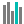#LOGNORM.INV (PQL - xl)

This function returns the inverse of the lognormal cumulative distribution function of x, where LN(x) is normally distributed with the specified mean and standard deviation

• Library: PQL \ Spreadsheet \ Statistical
• Compatibility: Any content (regardless of data source) in the Tabulate spreadsheet module
• Solve: This function can be used with the Solve plug-in: both as part of the objective function and constraint functions

#### Syntax

LOGNORM.INV(probability, mean, standard dev)

##### Function Arguments
 Name Description Type Optional probability Value at which to evaluate the function Number mean Value of mean of the natural logarithm of x, LN(x) Number standard dev Value representing the standard deviation of LN(x) Number